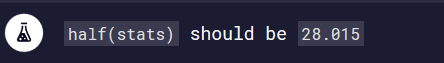# How does the function arrow understands which specific object i am referring too?

So i completed the challenge but basically cause i copied the logic from the description before.I cant understand how i can destructure inside the parenthesis and the arrow function understand in which object i am refering to take its properties.Lets say that there was another object with different name that had also 1 max and 1 min property.My arrow function in the code below how could know what to do?

``````
const stats = {
max: 56.78,
standard_deviation: 4.34,
median: 34.54,
mode: 23.87,
min: -0.75,
average: 35.85
};

// Only change code below this line
const half = ({max,min}) => (max + min) / 2.0;
// Only change code above this line}
``````

User Agent is: `Mozilla/5.0 (Windows NT 10.0; WOW64) AppleWebKit/537.36 (KHTML, like Gecko) Chrome/84.0.4147.125 Safari/537.36`.

Challenge: Use Destructuring Assignment to Pass an Object as a Function’s Parameters

Your function is going to destructure the object that you pass to it.

``````const obj1 = { a: 10, b: 20 };
const obj2 = { a: 15, b: 30 };

const sum = ({ a, b }) => a + b;
sum(obj1); // 30
sum(obj2); // 45
``````

Destructuring is basically shorthand, if we were to write the same code without destructuring it would look something like this:

``````const sum = (a, b) => a + b;
sum(obj1.a, obj1.b); // 30
sum(obj2.a, obj2.b); // 45
``````

So you see, the effect is the same but when we call the function we have to specify each argument, whereas when we use destructuring we can just pass an object.1 Like

The function doesn’t “know”. An object gets passed into the function as an argument when it called:1 Like